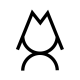# 114 Fluid Pressure: Aquarium PigxmDemo

The water exerts an upward pressure force on the card (F=P.A). If the pressure force is larger than the brass weight, then the card is pressed upward against the tube, and the pig is safe.

Since hydro-static pressure is proportional to the depth of the fluid (P = hρg), the pressure force decreases as the tube is raised slowly towards the water surface. There will come a point where the pressure force is less than the brass weight, the card drops away from the tube, and the pig is doomed.

Let’s do a rough calculation. The mass of the brass weights is 300 g. The inner radius of the tube is 8.6 cm.

P.A = mg

gr2 = mg

h(1000).(3.14)(0.0432) = 0.300

h = 5.17 cm

By equating the weight to the upward…

View original post 24 more words